# bp算法

2015/08/04 17:45

## BP神经网络理论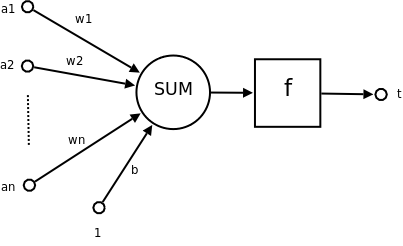• a1~an为输入向量的各个分量

• w1~wn为神经元各个突触的权值

• b为偏置（bias），或者称之为阈值（threshold）

• f为传递函数，通常为非线性函数。一般有traingd(),tansig(),hardlim()。以下默认为hardlim()

• t为神经元输出

数学表示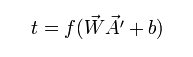• 向量W为权向量

• 向量A为输入向量，做内积需要用到转置

• b为偏置（bias），或者称之为阈值（threshold）

• f为传递函数，也即激励函数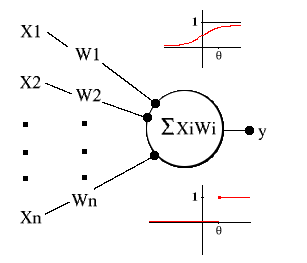• 向量W为权向量

• 向量X为输入向量

• b为偏置（bias），或者称之为阈值（threshold）

首先解释一下单个神经元如何进行分类判断：

引入一个阈值逻辑单元（Threshold Logic Unit，TLU）的名词，它可以输入一组加权系数的量，对它们进行求和，如果这个和达到或者超过了某个阈值，输出一个量。

a = (X1 * W1)+(X2 * W2)+...+(Xi * Wi)+...+ (Xn * Wn)

假 设一个 TLU 有两个输入值，它们的权系数等于 1，theta 值等于 1.5。当这个 TLU 输入  <0,0>、<0,1>、<1,0> 和 <1,1> 时，它的输出分别为 0、0、0、1。TLU  将这些输入分为两组：0 组和 1 组。就像懂得逻辑连接（布尔运算 AND）的人脑可以类似地将逻辑连接的句子分类那样，TLU  也懂得一点逻辑连接之类的东西。

神经网络的学习是模仿大脑调节神经网络连接的原理。TLU通过改变它的权值以及阈值来进行学习。回想上面，我们看到SUM()>=theta时候，TLU在临界点是输出的是1而不是0，这就相当于当SUM(Wi * Xi)  +  (-1 * theta) >= 0的时候。这时候，我们把-1当做是一个常量输入，而他的权系数theta θ在学习过程(或者说是训练过程)中进行调整。这样，当 SUM(X i* W i)+ (-1 * theta) >= 0 时，y=1，反之 y=0。

1. 一系列需要分类的术语示例X(input)

2. 它们的正确分类或者目标Target

 理想化的学习算法 fully_trained = FALSEDO UNTIL (fully_trained):    fully_trained = TRUE    FOR EACH training_vector = ::                               # Weights compared to theta        a = (X1 * W1)+(X2 * W2)+...+(Xn * Wn) - theta        y = sigma(a)        IF y != target:            fully_trained = FALSE        FOR EACH Wi:        MODIFY_WEIGHT(Wi)      # According to the training rule    IF (fully_trained):        BREAK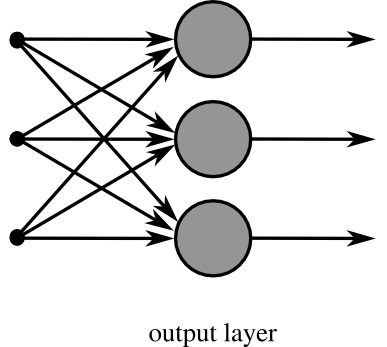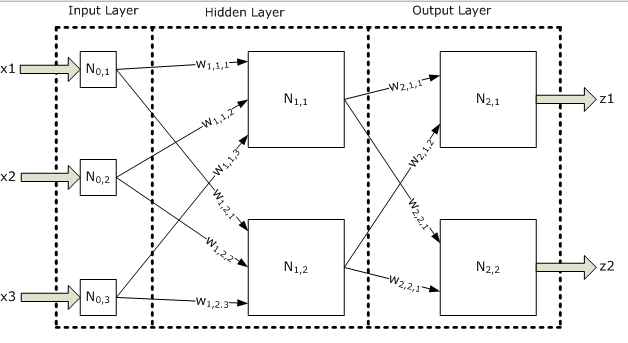BP (Back Propagation)神经网络，即误差反传误差反向传播算法的学习过程，由信息的正向传播和误差的反向传播两个过程组成。由上图可知，BP神经网络是一个三层的网络:

• 输入层(input layer)：输入层各神经元负责接收来自外界的输入信息，并传递给中间层各神经元；

• 隐藏层(Hidden Layer):中间层是内部信息处理层，负责信息变换，根据信息变化能力的需求，中间层可以设计为单隐层或者多隐层结构；最后一个隐层传递到输出层各神经元的信息，经进一步处理后，完成一次学习的正向传播处理过程；

• 输出层(Output Layer):顾名思义，输出层向外界输出信息处理结果；

当实际输出与期望输出不符时，进入误差的反向传播阶段。误差通过输出层，按误差梯度下降的方式修正各层权值，向隐层、输入层逐层反传。周而复始的信息正 向传播和误差反向传播过程，是各层权值不断调整的过程，也是神经网络学习训练的过程，此过程一直进行到网络输出的误差减少到可以接受的程度，或者预先设定 的学习次数为止。

好 了，以上对于神经网络的结构以及各个组件之间的运行时关系描述到此为止，那么，现在重要的是：具体是如何在误差反向传播的过程中，对各个层权系数进行调 整？规则是什么？总结上面，调整的目的即是每次训练后减少误差。可以使用最小二乘法或者是梯度下降算法。首先需要介绍一下Delta学习规则

 sum = 0FOR p = 1 TO M:         # M is number of training vectors    FOR n = 1 TO N:     # N is number of output nodes        sum = sum + (1/2 * (t(p,n)-y(p,n))^2)average = 1/M * sum

delta  规则就是依据这个误差的定义来定义的。因为误差是依据那些培训向量来说明的，delta  规则是一种获取一个特殊的权系数集以及一个特殊的向量的算法。而改变权系数将会使神经网络的误差最小化。我们不需要讨论支持这个算法的微积分学，只要认为 任何Wi发生的变化都是如下所示就够了：

alpha * s'(a(p,n)) * (t(p,n) - y(p,n)) * X(p,i,n)

X(p,i,n)是输入到节点n的p中的第i个元素，alpha是已知的学习率。最后 s'(a(p,n))是与p相关的第n个节点激活的 squashing 函数的变化（派生）率，这就是 delta 规则。

alpha * s'(a(p,n)) * d(n) * X(p,i,n)

1. n 对任何给出的输出节点有多大影响；

2. 输出节点本身对网络整体的误差有多少影响。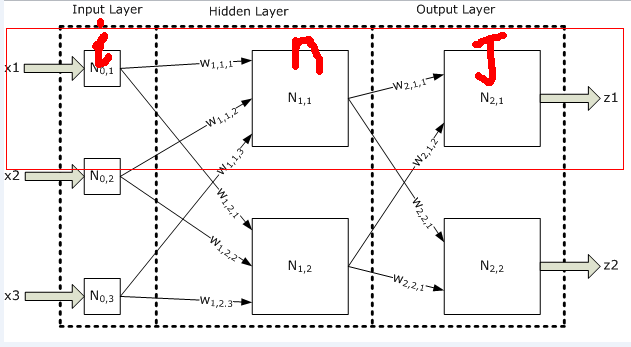SUM(d(j)*W(n,j))

alpha * s'(a(p,n))*(t(p,n) - y(p,n)) * X(p,n,j)

alpha * s'(a(p,n)) * sum(d(j) * W(n,j)) * X(p,i,n)

http://en.wikipedia.org/wiki/Backpropagation wiki上面的BP介绍

0
4 收藏

### 作者的其它热门文章0 评论
4 收藏
0